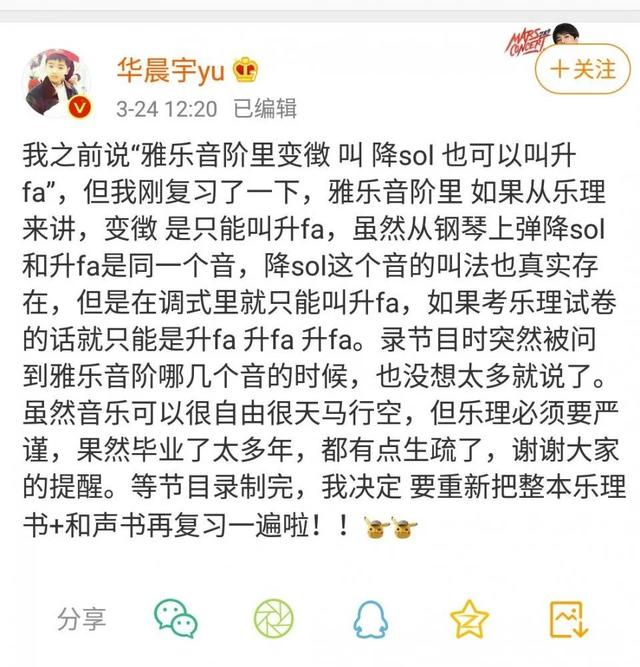# 音乐 用 Ruby 学习基本乐理（二）：音程

dsh0416 · 2020年04月11日 · 最后由 dsh0416 回复于 2020年05月06日 · 4853 次阅读

## 音数

``````class Tone
STANDARD_TUNING = 440.0

def initialize(name)
@name = name
@offset = parse_name(name)
@frequency = frequency_by_offset(@offset)
end

def parse_name(name)
raise AugumentError unless name.match?(/^[CDEFGAB][#,b]*\d\$/)
tone = name
sharps = name[1...-1]
range = name[-1].to_i

# Calculate offset
major_scale = [-9, -7, -5, -4, -2, 0, 2]
offset = major_scale['CDEFGAB'.index(tone)] + (range - 4) * 12 # Offset without sharps or flats

sharps.chars.each do |c|
c == '#' ? offset += 1 : offset -= 1
end

offset
end

def frequency_by_offset(offset)
STANDARD_TUNING * (2.0 ** (1.0 / 12)) ** offset
end

def harmonic_series(max=Tone.new('B9').frequency)
tones = []
(2..).each do |n|
break if @frequency * n > max
tones << Tone.by_frequency(@frequency * n)
end
tones.uniq { |t| t.name }
end

def -(tone)
raise TypeError unless tone.is_a?(Tone)
@offset - tone.offset
end

class << self
ALL_TONES = (0..9).map {|range| ['C', 'C#', 'D', 'D#', 'E', 'F', 'F#', 'G', 'G#', 'A', 'A#', 'B'].map {|name| "#{name}#{range}"}}.flatten.map { |name| Tone.new(name) }

def by_frequency(freq)
errs = ALL_TONES.map {|x| (x.frequency - freq).abs }
ALL_TONES[errs.rindex(errs.min)]
end
end
end

p Tone.new('C3') - Tone.new('D3') # => -2
``````

## 音级（这下理解流行乐坛乐理小王子怎么在节目里讲乐理被真音乐学院副教授给骂了吧。）

C 1 2 3 4 5 6 7
D 2 1 2 3 4 5 6
E 3 2 1 2 3 4 5
F 4 3 2 1 2 3 4
G 5 4 3 2 1 2 3
A 6 5 4 3 2 1 2
B 7 6 5 4 3 2 1

``````def interval_number(tone)
raise TypeError unless tone.is_a?(Tone)
if self - tone > 0
'CDEFGAB'.index(@name) - 'CDEFGAB'.index(tone.name) + (@name[-1].to_i - tone.name[-1].to_i) * 7 + 1
else
'CDEFGAB'.index(tone.name) - 'CDEFGAB'.index(@name) + (tone.name[-1].to_i - @name[-1].to_i) * 7 + 1
end
end

p Tone.new('C3').interval_number(Tone.new('D3')) # => 2
p Tone.new('C3').interval_number(Tone.new('G2')) # => 4
p Tone.new('C3').interval_number(Tone.new('C1')) # => 15
``````

## 形态

``````def interval_type(tone)
raise TypeError unless tone.is_a?(Tone)
semitones = (self - tone).abs % 12
number = (interval_number(tone) - 1) % 7 # Eighth's type is equal to union's.
default_type_semitones = [0, 2, 4, 5, 7, 9, 11]
default_types = %w(P M M P P M M)
delta = semitones - default_type_semitones[number]
current_type = default_types[number]

until delta == 0
if delta > 0 and (current_type == 'P' or current_type == 'M')
delta -= 1
current_type = 'A'
elsif delta > 0
delta -= 1
current_type = "A#{current_type}"
elsif delta < 0 and (current_type == 'P' or current_type == 'm')
delta += 1
current_type = "d"
else
delta += 1
current_type = "d#{current_type}"
end
end

current_type
end

p Tone.new('C3').interval_type(Tone.new('D3')) # => "M"
p Tone.new('C3').interval_type(Tone.new('G2')) # => "P"
p Tone.new('C3').interval_type(Tone.new('C1')) # => "P"
``````

## 总结

``````def interval(tone)
"#{interval_type(tone)}#{interval_number(tone)}"
end

p Tone.new('C3').interval(Tone.new('D3')) # => "M2"
p Tone.new('C3').interval(Tone.new('G2')) # => "P4"
p Tone.new('C3').interval(Tone.new('C1')) # => "P15"
p Tone.new('C3').interval(Tone.new('D####3')) # => "AAAA2"
``````

1 楼 已删除